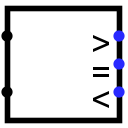#Komparator

 Bibliothek: Arithmetic Eingeführt: 2.0 Beta 22 Aussehen:## Verhalten

Compares two values, either as unsigned values or as two's-complement values, depending on the Numeric Type attribute. Normally, one of the outputs will be 1, and the other two outputs will be 0.

The comparison is performed starting at the most significant bits in each number and descending downward in parallel until a location is found where the two values disagree. If, however, an error value or a floating value is encountered during this descent, then all outputs will match that error or floating value.

## Pins

West edge, north end (input, bit width matches Data Bits attribute)
The first of the two values to be compared.
West edge, south end (input, bit width matches Data Bits attribute)
The second of the two values to be compared.
East edge, labeled > (output, bit width 1)
1 if the first input is greater than the second input, 0 if the first input is less than or equal the second input.
East edge, labeled = (output, bit width 1)
1 if the first input equals the second input, 0 if the first input is not equal the second input.
East edge, labeled < (output, bit width 1)
1 if the first input is less than the second input, 0 if the first input is greater than or equal the second input.

## Attribute

When the component is selected or being added, Alt-0 through Alt-9 alter its Data Bits attribute.

Data Bits
The bit width of the component's inputs and outputs.

Keines.

## Verhalten des Textwerkzeugs

Keines.

Zurück zur Bibliotheksreferenz